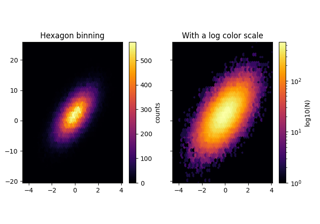# matplotlib.axes.Axes.hexbin¶

Axes.hexbin(self, x, y, C=None, gridsize=100, bins=None, xscale='linear', yscale='linear', extent=None, cmap=None, norm=None, vmin=None, vmax=None, alpha=None, linewidths=None, edgecolors='face', reduce_C_function=<function mean at 0x7fcc1b66a310>, mincnt=None, marginals=False, *, data=None, **kwargs)[source]

Make a 2D hexagonal binning plot of points x, y.

If C is None, the value of the hexagon is determined by the number of points in the hexagon. Otherwise, C specifies values at the coordinate (x[i], y[i]). For each hexagon, these values are reduced using reduce_C_function.

Parameters:
x, yarray-like

The data positions. x and y must be of the same length.

Carray-like, optional

If given, these values are accumulated in the bins. Otherwise, every point has a value of 1. Must be of the same length as x and y.

gridsizeint or (int, int), default: 100

If a single int, the number of hexagons in the x-direction. The number of hexagons in the y-direction is chosen such that the hexagons are approximately regular.

Alternatively, if a tuple (nx, ny), the number of hexagons in the x-direction and the y-direction.

bins'log' or int or sequence, default: None

Discretization of the hexagon values.

• If None, no binning is applied; the color of each hexagon directly corresponds to its count value.
• If 'log', use a logarithmic scale for the color map. Internally, $$log_{10}(i+1)$$ is used to determine the hexagon color. This is equivalent to norm=LogNorm().
• If an integer, divide the counts in the specified number of bins, and color the hexagons accordingly.
• If a sequence of values, the values of the lower bound of the bins to be used.
xscale{'linear', 'log'}, default: 'linear'

Use a linear or log10 scale on the horizontal axis.

yscale{'linear', 'log'}, default: 'linear'

Use a linear or log10 scale on the vertical axis.

mincntint > 0, default: None

If not None, only display cells with more than mincnt number of points in the cell.

marginalsbool, default: False

If marginals is True, plot the marginal density as colormapped rectangles along the bottom of the x-axis and left of the y-axis.

extentfloat, default: None

The limits of the bins. The default assigns the limits based on gridsize, x, y, xscale and yscale.

If xscale or yscale is set to 'log', the limits are expected to be the exponent for a power of 10. E.g. for x-limits of 1 and 50 in 'linear' scale and y-limits of 10 and 1000 in 'log' scale, enter (1, 50, 1, 3).

Order of scalars is (left, right, bottom, top).

Returns:
PolyCollection

A PolyCollection defining the hexagonal bins.

If marginals is True, horizontal bar and vertical bar (both PolyCollections) will be attached to the return collection as attributes hbar and vbar.

Other Parameters:
cmapstr or Colormap, default: rcParams["image.cmap"] (default: 'viridis')

The Colormap instance or registered colormap name used to map the bin values to colors.

normNormalize, optional

The Normalize instance scales the bin values to the canonical colormap range [0, 1] for mapping to colors. By default, the data range is mapped to the colorbar range using linear scaling.

vmin, vmaxfloat, default: None

The colorbar range. If None, suitable min/max values are automatically chosen by the Normalize instance (defaults to the respective min/max values of the bins in case of the default linear scaling). It is deprecated to use vmin/vmax when norm is given.

alphafloat between 0 and 1, optional

The alpha blending value, between 0 (transparent) and 1 (opaque).

linewidthsfloat, default: None

If None, defaults to 1.0.

edgecolors{'face', 'none', None} or color, default: 'face'

The color of the hexagon edges. Possible values are:

• 'face': Draw the edges in the same color as the fill color.
• 'none': No edges are drawn. This can sometimes lead to unsightly unpainted pixels between the hexagons.
• None: Draw outlines in the default color.
• An explicit color.
reduce_C_functioncallable, default: numpy.mean

The function to aggregate C within the bins. It is ignored if C is not given. This must have the signature:

def reduce_C_function(C: array) -> float


Commonly used functions are:

**kwargsPolyCollection properties

All other keyword arguments are passed on to PolyCollection:

Property Description
agg_filter a filter function, which takes a (m, n, 3) float array and a dpi value, and returns a (m, n, 3) array
alpha float or None
animated bool
antialiased or aa or antialiaseds bool or list of bools
array ndarray
capstyle {'butt', 'round', 'projecting'}
clim (vmin: float, vmax: float)
clip_box Bbox
clip_on bool
clip_path Patch or (Path, Transform) or None
cmap Colormap or str or None
color color or list of rgba tuples
contains unknown
edgecolor or ec or edgecolors color or list of colors or 'face'
facecolor or facecolors or fc color or list of colors
figure Figure
gid str
hatch {'/', '\', '|', '-', '+', 'x', 'o', 'O', '.', '*'}
in_layout bool
joinstyle {'miter', 'round', 'bevel'}
label object
linestyle or dashes or linestyles or ls str or tuple or list thereof
linewidth or linewidths or lw float or list of floats
norm Normalize or None
offset_position unknown
offsets array-like (N, 2) or (2,)
path_effects AbstractPathEffect
picker None or bool or callable
pickradius unknown
rasterized bool or None
sketch_params (scale: float, length: float, randomness: float)
snap bool or None
transform Transform
url str
urls list of str or None
visible bool
zorder float

Notes

Note

In addition to the above described arguments, this function can take a data keyword argument. If such a data argument is given, the following arguments can also be string s, which is interpreted as data[s] (unless this raises an exception): x, y.

Objects passed as data must support item access (data[s]) and membership test (s in data).

## Examples using matplotlib.axes.Axes.hexbin¶